# Plus Two Maths Chapter Wise Questions and Answers Chapter 12 Linear Programming

Students can Download Chapter 12 Linear Programming Questions and Answers, Plus Two Maths Chapter Wise Questions and Answers helps you to revise the complete Kerala State Syllabus and score more marks in your examinations.

## Kerala Plus Two Maths Chapter Wise Questions and Answers Chapter 12 Linear Programming

### Plus Two Maths Linear Programming Four Mark Questions and Answers

Question 1.
Solve the following LPP Graphically;
Maximise; Z = 60x + 15y
Subject to constraints;
x + y ≤ 50, 3x + y ≤ 90, x ≥ 0, y ≥ 0.
1. In the figure the shaded region OABC is the fesible region. Here the region is bounded. The corner points are O(0, 0), A(30, 0), B(20, 30), C(0, 50).Given; Z = 60x + 15y

 Corner points Value of Z O Z = 0 A Z = 60(30) + 15(0) = 1800 B Z = 60(20) + 15(30) = 1650 C Z = 60(0) + 15(50) = 750

Since maximum value of Z occurs at A, the soluion is Z = 1800, (30, 0).Question 2.
Solve the following LPP Graphically;
Minimise; Z = -3x + 4y
Subject to constraints;
x + 2y ≤ 8, 3x + 2y ≤ 12, x ≥ 0, y ≥ 0
In the figure the shaded region OABC is the fesible region. Here the region is bounded. The corner points are O(0, 0), A(4, 0) B(2, 3), C(0, 4).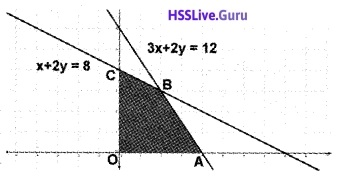Given; Z = -3x + 4y

 Corner points Value of Z O Z = 0 A Z = -3(4) + 4(0) = -12 B Z = -3(2) + 4(3) = 6 C Z = -3(0) + 4(4) = 16

Since minimum value of Z occurs at A, the soluion is Z = -12, (4, 0).Question 3.
Solve the following LPP Graphically;
Maximise; Z = 3x + 5y
Subject to constraints;
x + 3y ≥ 3, x + y ≥ 2, x ≥ 0, y ≥ 0
In the figure the shaded region ABC is the fesible region. Here the region is unbouded.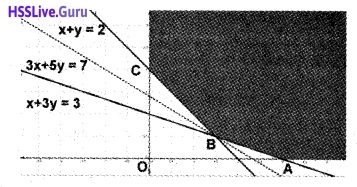The corner points are A(3, 0), B$$\left(\frac{3}{2}, \frac{1}{2}\right)$$, C(0, 2)
Given; Z = 3x + 5y

 Corner points Value of Z A Z = 3(3) + 5(0) = 9 B Z = 3$$\left(\frac{3}{2}\right)$$ + 5$$\left(\frac{1}{2}\right)$$ = 7 C Z = 3(0) + 5(2) = 10

Form the table, minumum value of Z is 7 at B$$\left(\frac{3}{2}, \frac{1}{2}\right)$$. The feasible region is unbounded, so consider the inequality 3x + 5y < 7. Clearly the feasible region has no common points with 3x + 5y < 7, Thus minimum value of Z occurs at B, the soluion is Z = 7.

### Plus Two Maths Linear Programming Six Mark Questions and Answers

Question 1.
One kind of a cake requires 200g of flour and 25g of fat, and another kind of cake requires 100g of flour and 50g of fat. Find the maximum number of cakes which can be made from 5kg of flour and 1kg of fat assuming that there is no shortage of the other ingredients, used in making the cake.
Let the number of cakes made of type I are x and that of type II are y. Then the total number of cakes will be Z = x + y
Flour constraint 200x + 100y ≤ 5000
Fat constraint 25x + 50y ≤ 1000
Therefore;
Maximise; Z = x + y
2x + y ≤ 50; x + 2y ≤ 40; x ≥ 0, y ≥ 0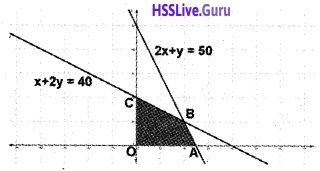In the figure the shaded region OABC is the feasible region. Here the region is bounded. The corner points are O(0, 0), A(25, 0), B(20, 10), C(0, 20)
Given; Z = x + y

 Corner points Value of Z O Z = 0 A Z = 25 + 0 = 25 B Z = 20 + 10 = 30 C Z = 0 + 20 = 20

Since maximum value of Z occurs at B, the soluion is Z = 30, (20, 10).Question 2.
A factory makes tennis rackets and cricket bats. A tennis racket takes 1.5 hours of machine and 3hours of craftman’s time in its making, while a cricket bat takes 3 hours of machine time and 1 hour of craftman’s time. In a day, the factory has availability of not more than 42 hours of machine time and 24 hours of craftman’s time.

1. What no. of rackets and bats must be produced if the factory is to work at full capacity?
2. If the profit on a racket and a bat is 10 find maximum profit.

Let the number of rackets made = x and that of bats = y.
Maximise; Z = x + y
Machine constraints 1.5x + 3y ≤ 42
Craftsman’s constraint 3x + y ≤ 24
Therefore; Maximise; Z = x + y
x + 2y ≤ 14, 3x + y ≤ 24, x ≥ 0, y ≥ 0
In the figure the shaded region OABC is the fesible region. Here the region is bounded. The corner points are O(0, 0), A(8, 0), B(4, 10), C(0, 14).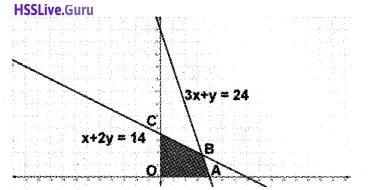Given; Z = x + y

 Corner points Value of Z O Z = 0 A Z = 8 + 0 = 8 B Z = 4 + 12 = 16 C Z = 0 + 14 = 14

Since maximum value of Z occurs at B, the soluion is Z = 16, (4, 12).Question 3.
Two godowns A and B have grains capacity of 100 quintals and 50 quintals respectively. They supply to 3 ration shops D, E, and F whose requirement are 60, 50 and 40 quintals respectively. The cost of transportation per quintal from the godowns to the shops is given in the following table; Transportation cost per quintal(in Rs.)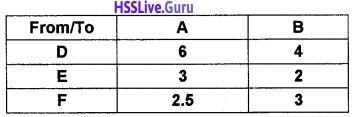Hence should the supplies be transported in order that the transportation cost is minimum? What is the minimum cost?Express the problem diagrammatically as shown above. The total transportation cost is given by
Z = 6x + 3y + 2.5{100 – (x + y)} + 4(60 – x) + 2(50 – y) + 3(-60 + (x + y))
⇒ Z = 2.5x + 1.5y + 410
100 – (x + y) ≥ 0 ⇒ x + y ≤ 100
60 – x ≥ 0 ⇒ x ≤ 60
50 – y ≥ 0 ⇒ y ≤ 50 – 60 + x + y ≥ 0 ⇒ x + y ≥ 60
Then the given LPP is
Minimise; Z = 2.5x + 1.5y + 410
x + y ≤ 100, x + y ≥ 60
0 ≤ x ≤ 60, 0 ≤ y ≤ 50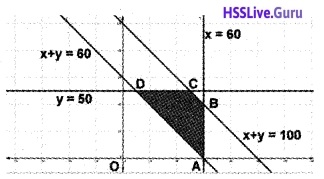In the figure the shaded region ABCD is the feasible region. Here the region is bounded. The corner points are
A(60, 0), B(60, 40), C(50, 50), D(10, 50).
Given; Z = 2.5x + 1.5y + 410

 Corner points Value of Z A Z = 2.5(60) + 1.5(0) + 410= 560 B Z = 2.5(60) + 1.5(40) + 410 = 620 C Z = 2.5(50) + 1.5(50) + 410 = 610 D Z = 2.5(10) + 1.5(50) + 410 = 510

Since minimum value of Z occurs at D, the soluion is Z = 510.Question 4.
(i) Choose the correct answer from the bracket. If an LPP is consistent, then its feasible region is always
(a) Bounded
(b) Unbounded
(c) Convex region
(d) Concave region
(ii) Maximize Z = 2x + 3y subject to the constraints x + y ≤ 4, x ≥ 0, y ≥ 0.
(i) (c) Convex region.

(ii)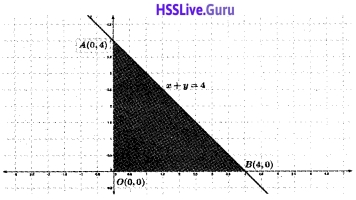Corner points of the feasible region are as follows

 Corner points Z = 2x + 3y 0(0, 0) 0 A(0, 4) 12 → Maximum B(4, 0) 8

∴ the maximum value of Z is 12 attained at (0, 4).Question 5.
The graph of a linear programming problem is given below. The shaded region is the feasible region. The objective function is Z = px + qy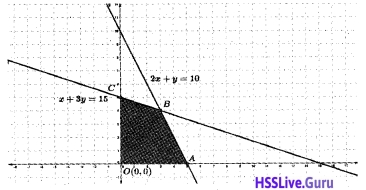1. What are the co-ordinates of the comers of the feasible region.
2. Write the constraints
3. If the Max. Z occurs at A and B, what is the relation between p and q?
4. If q = 1, write the objective function
5. Find the Max Z

1. Corner points are O(0, 0), A(5, 0), B(3, 4), C(0, 5).

2. Constraints are 2x + y ≤ 10, x + 3y ≤ 15, x ≥ 0, y ≥ 0.

3. At (3, 4), Z = 3p + 4q
At (5, 0), Z = 5p
⇒ 3p + 4q = 5p ⇒ p = 2q.

4. If q = 1, p=2
Then the objective function is,
Maximize Z = 2x + y.

5. At (3, 4) Z = 2 × 3 + 4 = 10 is the maximum value.Question 6.
A diet is to contain at least 80 units of vitamin A and 100 units of minerals. Two foods F1 and F2 are available. Food F1 costs Rs 4 per unit food and F2 costs Rs 6 per unit. One unit of food F1 contains 3 units of vitamin A and 4 units of minerals. One unit of food F2 contains 6 units of vitamin A and 3 units of minerals. Formulate this as a linear programming problem. Find the minimum costs for diet that consists of mixture of these two foods and also meets the minimal nutritional requirements.
Let x units of food F1 and y units of food F2 be in the diet
Total cost Z = 4x + 6y
Then the LPP is
Minimize Z = 4x + 6
Subject to the constraints
3x + 6y ≥ 80
4x + 3y ≥ 100
x, y ≥ 0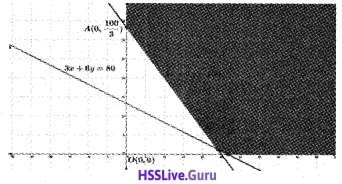The feasible region is unbounded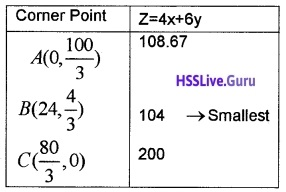As the feasible region is unbounded, 104 may or may not be the minimum value of Z. For this we draw a graph of the inequality 4x + 6y < 104 or 2x + 3y < 52 and check whether the resulting half plane has points in common with the feasible region or not.

It can be seen that the feasible region has no common points with 2x + 3y < 52 Therefore minimum cost of the mixture will be 104.